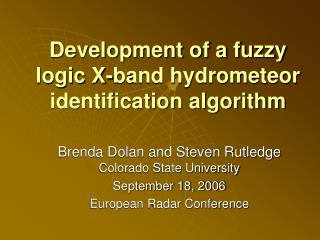DownloadDownload PresentationDevelopment of a fuzzy logic X-band hydrometeor identification algorithm

# Development of a fuzzy logic X-band hydrometeor identification algorithm

Download Presentation## Development of a fuzzy logic X-band hydrometeor identification algorithm

- - - - - - - - - - - - - - - - - - - - - - - - - - - E N D - - - - - - - - - - - - - - - - - - - - - - - - - - -
##### Presentation Transcript

1. Development of a fuzzy logic X-band hydrometeor identification algorithm Brenda Dolan and Steven RutledgeColorado State University September 18, 2006 European Radar Conference

2. Road Map • Motivation and Background • Methodology • Simulations using T-matrix and Mueller matrix • Results • Future work

3. Introduction • Collaborative Adaptive Sensing of the Atmosphere (CASA) Integrated project (IP1) • Network of 4 polarimetric X-band radars located in Oklahoma • Analyze the microphysical and kinematic structure of storms observed by the CASA IP1 network • Dual-Doppler analysis • Polarimetric analysis • Hydrometeor ID (HID) • Rain rate /snowfall estimation

4. Introduction • Why is radar identification of hydrometeors important? • Identify regions of mixed phase • Determine possible regions of freezing rain and sleet • Use to drive rainrate and snowfall algorithms • Identify locations of possible ice contamination • Distinguish between aggregates and ice crystals • Locate large hail • Help validate numerical models Dolan and Rutledge, 2006

5. S-band vs. X-band • Extensive research and algorithm development at S-band (Straka et al. 2000, Liu and Chandrasekar 2001, Tessendorf et al. 2005) • Include observational data • Extending into C-band • Little work has been done on HID algorithms for X-band • Why do we expect the HID algorithm for X-band and S-band to be different? • Mie scattering • Resonance • Attenuation • Kdp proportional to 1/l (Bringi and Chandrasekar 2001, Matrosov et al. 2006)

6. Fuzzy Logic • One approach to HID is using fuzzy logic • Combine information from polarimetric variables, as well as temperature • Zh, Zdr, Kdp, rhv in this case (no Ldr) • Soft boundaries and ranges for each of the variables • Variables can be weighted • Each hydrometeor type is given a score based on how well the variables fit into the fuzzy set • Hydrometeor with highest score is considered the hydrometeor type at that data point

7. a b m Fuzzy Logic: Membership Beta Functions (MBFs) Zh Zdr Kdp LDR Zh ρhv These MBFs are from Tessendorf et al. (2005) for S-band T

8. Hydrometeor Types • We are interested in distinguishing the following precipitation types: • Rain • Drizzle • Wet (low-density) graupel • Dry (high-density) graupel • Snow (wet) • Snow (dry) • Pristine ice crystals (dendrites/columns) • Vertically aligned ice crystals • Hail (added complications)

9. Hydrometeor Types • We are interested in distinguishing the following precipitation types: • Rain • Drizzle • Wet (low-density) graupel • Dry (high-density) graupel • Snow (wet) • Snow (dry) • Pristine ice crystals (dendrites/columns) • Vertically aligned ice crystals • Hail (added complications)

10. Methodology • Determine the “most probable” ranges of variables at X-band • Thus determining m (midpoint) and a (width) of MBF • Using T-matrix and Mueller Matrix (Barber and Yeh 1975, Vivekanandan et al. 1991) scattering models to simulate a variety of precipitation events • Provides simulated radar data • Assign a “confidence” to the determined ranges => b (MBF slope) • As the algorithm is tested, modify these to become “more confident” about data ranges • Run simulations for both X and S-band (for comparison with literature)

11. Methodology: T-matrix • T-matrix: Simulates the scattering properties of a single particle • Can change: • Radar wavelength (3.2 cm, 11.0 cm) • Particle input sizes (rain: 0.05 cm to 1.0 cm, drizzle: 0.1 cm-0.6 cm) • Temperature (-10, 0, 10, 20 ºC) • Size-Axis ratio relationship (Goddard and Cherry, 1984) • Dielectric constant • Follow parameters for different hydrometeors outlined in Straka et al. (2000) • Run the model changing a single parameter at a time

12. Methodology: Mueller Matrix • Mueller Matrix: Generates the radar observables for a simulated “radar volume” • Can change • Radar elevation angle (1.0, 30.0º) • Drop size distribution (DSD) (Do, No) • Canting angles (distribution and std dev) (0-10º) • Range of particle diameters to use • Base the parameters on Straka et al. (2000)

13. Methodology: Mueller Matrix • Run the model for a wide variety of DSDs • Use Marshall Palmer for rain • N(D)=N0exp- D • Fix No at 80,000 m3 cm-1 • Vary  as a function of rain rate •  =4.1 R-0.21 • R=1-200 mm hr-1 for rain • And assume: • Do=3.67 /  • Use monodisperse DSD for drizzle • R=0.1- 2 mm hr-1

14. Results: Rain dBz Zdr Kdp hv 1.00 6 20 60 0.95 25 0 0 Blue: simulated X-band results Green : simulated S-band results Red : Straka et al. 2000 (S00) S-band values (includes observational data)

15. Results: Drizzle dBz Zdr Kdp hv 1.00 30 0.8 0.08 0.0 0 0.0 0.96 0.00 Blue: simulated X-band results Green : simulated S-band results Red : Straka et al. 2000 (S00) S-band values (includes observational data)

16. Results: Key differences between S-band and X-band • For rain: Variables seem to be a bit higher at X-band over S-band • Especially Kdp • We expect this with wavelength scaling • For drizzle: Zdr and Kdp were higher for X- band over S-band

17. Future work • Resolve differences between S-band simulations and observations • Run simulations for • Rain (using Gamma distribution) • Graupel • Sleet • Different ice crystals and snow types • Test new X-band HID against S-band using observations • GPM Front Range Pilot Project • Implement in CASA radar network • Inter-compare with KOUN

18. Results